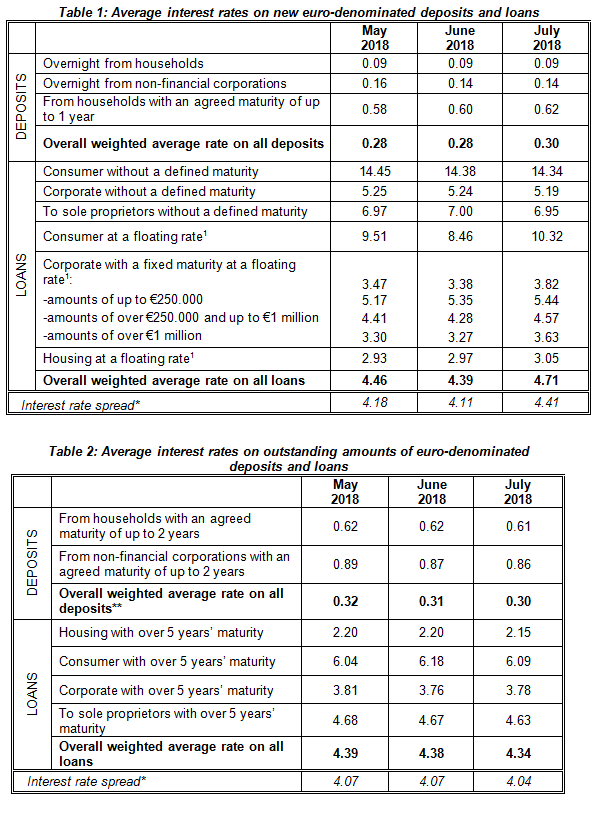# Press Releases

## Interest Rates on Bank Deposits and Loans: July 2018

### 03/09/2018 - Press Releases

1. INTEREST RATES ON EURO-DENOMINATED NEW DEPOSITS AND LOANS

In July 2018, the overall weighted average interest rate on new deposits remained almost unchanged, whereas the overall weighted average interest rate on new loans increased. The spread* between loan and deposit interest rates increased to 4.41 percentage points (see Table 1).

The overall weighted average interest rate on all new deposits remained almost unchanged at 0.30%. In particular, the average interest rates on overnight deposits placed by households and by non-financial corporations remained unchanged at 0.09% and 0.14%, respectively. The average interest rate on deposits from households with an agreed maturity of up to 1 year remained almost unchanged at 0.62%.

The overall weighted average interest rate on all new loans to households and non-financial corporations increased by 32 basis points to 4.71%.

More specifically, the average interest rate on consumer loans without a defined maturity (a category which comprises credit cards, open account loans and overdrafts) decreased by 4 basis points to 14.34%. The average interest rate on consumer loans with a defined maturity at a floating rate (1) increased by 186 basis points to 10.32%.

The average interest rate on corporate loans without a defined maturity decreased by 5 basis points to 5.19%. Τhe corresponding rate on loans to sole proprietors decreased also by 5 basis points to 6.95%. The average interest rate on corporate loans with a defined maturity at a floating rate (1) increased by 44 basis points to 3.82%. In particular, the rate on loans of up to €250,000 increased by 9 basis points to 5.44%, on loans of over €250,000 and up to €1 million increased by 29 basis points to 4.57%, while the rate on loans of over €1 million increased by 36 basis points to 3.63%. Finally, the average interest rate on housing loans at a floating rate (1) increased by 8 basis points to 3.05%.

2. INTEREST RATES ON OUTSTANDING AMOUNTS OF EURO-DENOMINATED DEPOSITS AND LOANS

In July 2018, the overall weighted average interest rate on the outstanding amounts of all deposits remained almost unchanged, while the corresponding rate on all loans decreased. The spread* between loan and deposit rates decreased to 4.04 percentage points (see Table 2).

In more detail, the overall weighted average interest rate on the outstanding amounts of all deposits (including overnight deposits) remained almost unchanged at 0.30%, while the corresponding rate on all loans decreased by 4 basis points to 4.34%.

In particular, the average interest rate on the outstanding amounts of deposits with an agreed maturity of up to 2 years placed by households remained almost unchanged at 0.61%. The average interest rate on the outstanding amounts of deposits with an agreed maturity of up to 2 years placed by non-financial corporations remained also almost unchanged at 0.86%. The average interest rate on the outstanding amounts of housing loans with over 5 years’ maturity decreased by 5 basis points to 2.15%. The average interest rate on the outstanding amounts of consumer loans (2) with over 5 years’ maturity decreased by 9 basis points to 6.09%. The corresponding rate on corporate loans remained almost unchanged at 3.78%. The average interest rate on the outstanding amounts of loans to sole proprietors with over 5 years’ maturity decreased by 4 basis points to 4.63%.*The interest rate spread is the difference between the overall weighted average rate on all loans and the overall weighted average rate on all deposits.
**For the calculation of the overall weighted average rate on all deposits the overnight deposits are also taken into account.

The complete data set of bank deposit and loan interest rates are published on the website of the Bank of Greece and can be accessed via the following link:
http://www.bankofgreece.gr/Pages/en/Statistics/rates_markets/deposits.aspx

Note: The next Press Release on “Interest Rates on Bank Deposits and Loans” for August 2018 will be published on 4 October 2018.

(1) Loans at a floating rate comprise also loans with an initial rate fixation period of up to one year.
(2) Including other loans to individuals and private non-profit institutions